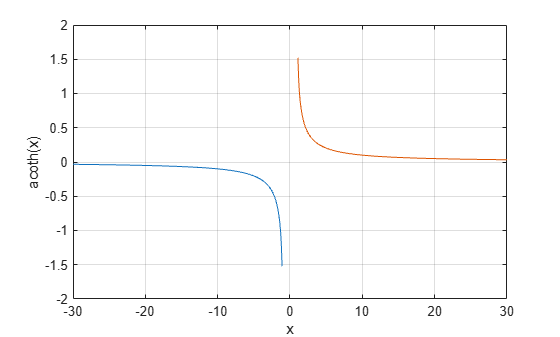# acoth

Inverse hyperbolic cotangent

## Syntax

``Y = acoth(X)``

## Description

example

````Y = acoth(X)` returns the inverse hyperbolic cotangent of the elements of `X`. The function accepts both real and complex inputs. All angles are in radians.```

## Examples

collapse all

Find the inverse hyperbolic cotangent of the elements of vector `X`. The `acoth` function acts on `X` element-wise.

```X = [2 -3 1+2i]; Y = acoth(X)```
```Y = 1×3 complex 0.5493 + 0.0000i -0.3466 + 0.0000i 0.1733 - 0.3927i ```

Plot the inverse hyperbolic cotangent function over the intervals $-30\le x<-1$ and $1.

```x1 = -30:0.1:-1.1; x2 = 1.1:0.1:30; plot(x1,acoth(x1),x2,acoth(x2)) grid on xlabel('x') ylabel('acoth(x)')```## Input Arguments

collapse all

Hyperbolic cotangent of angle, specified as a scalar, vector, matrix, or multidimensional array. The `acoth` operation is element-wise when `X` is nonscalar.

Data Types: `single` | `double`
Complex Number Support: Yes

collapse all

### Inverse Hyperbolic Cotangent

For real values $x$ in the domain $-\text{\hspace{0.17em}}\infty and $1, the inverse hyperbolic cotangent satisfies

`${\mathrm{coth}}^{-1}\left(x\right)={\mathrm{tanh}}^{-1}\left(\frac{1}{x}\right)=\frac{1}{2}\mathrm{log}\left(\frac{x+1}{x-1}\right).$`

For complex numbers $z=x+iy$ as well as real values in the domain $-1\le z\le 1$, the call `acoth(z)` returns complex results.